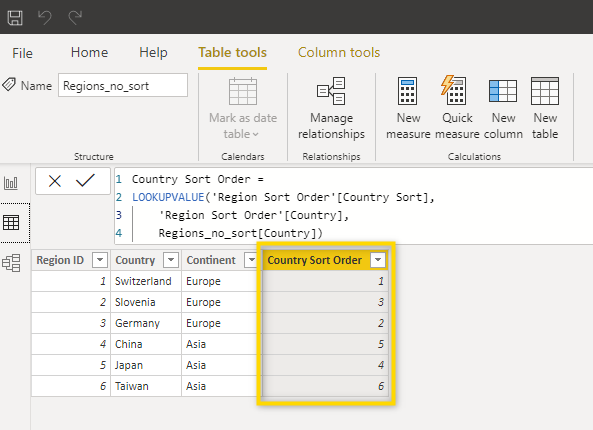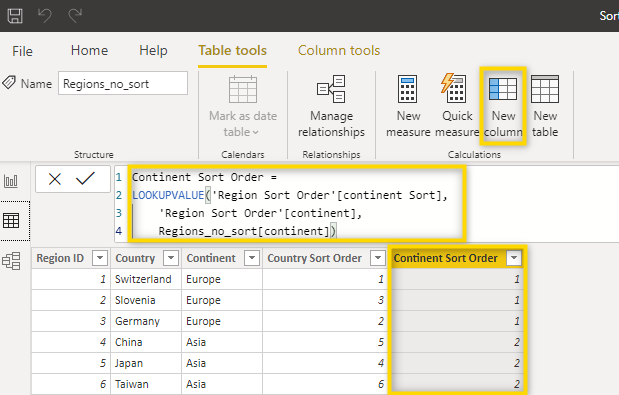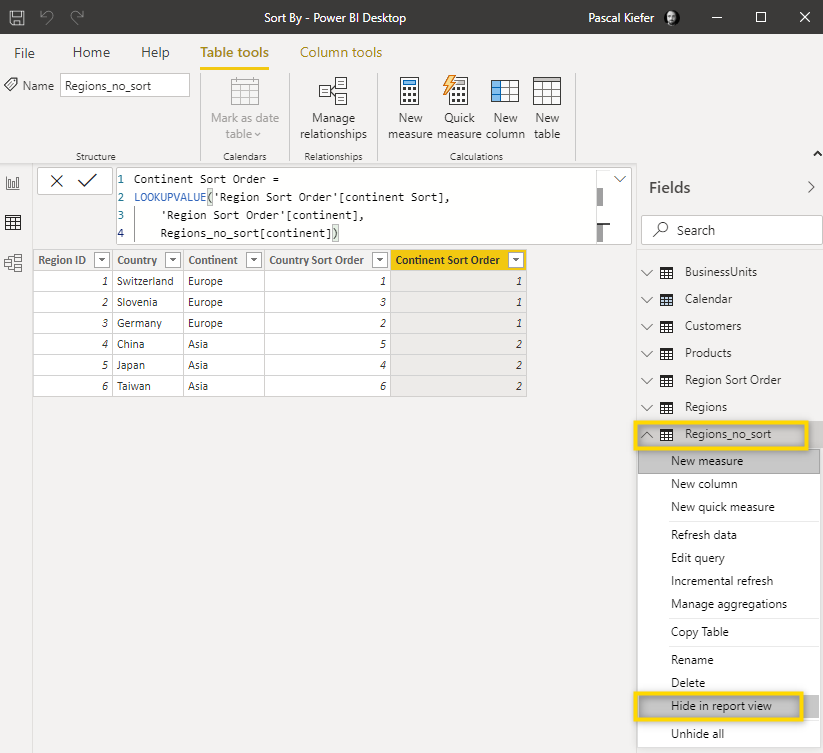# How to manually add a sorting column

### To set up a custom sort order logic, you must have a column with a numeric value. In case this column is not available in the data source, it has to be created manually.

This Knowledge Base contains some articles which explain how you can set up a custom sort order logic. This article shows how to sort by months and this one how to sort a hierarchy. In all cases, you need to have a column with the numeric value to sort by.

If your source system is Excel, then it is easy to just add a column which indicates the sort order. But you might be loading from a system that simply doesn't include the sort order and it would be too complicated to add it. Of course, we have a solution for this case. Let's see how you can manually add the sort order column.

Let's assume you want to sort a Continent/Country hierarchy in a specific way but no sorting columns are available. This is our data: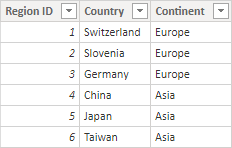And this is the sort order we want to see:

Europe

Switzerland

Germany

Slovenia

Asia

Japan

China

Taiwan

First of all, let's create a new table that will contain the custom sort order. To create a new manual table, select Enter data, fill out the table, give it a name, and click Load. You might as well create this table in Excel and load it into the data model.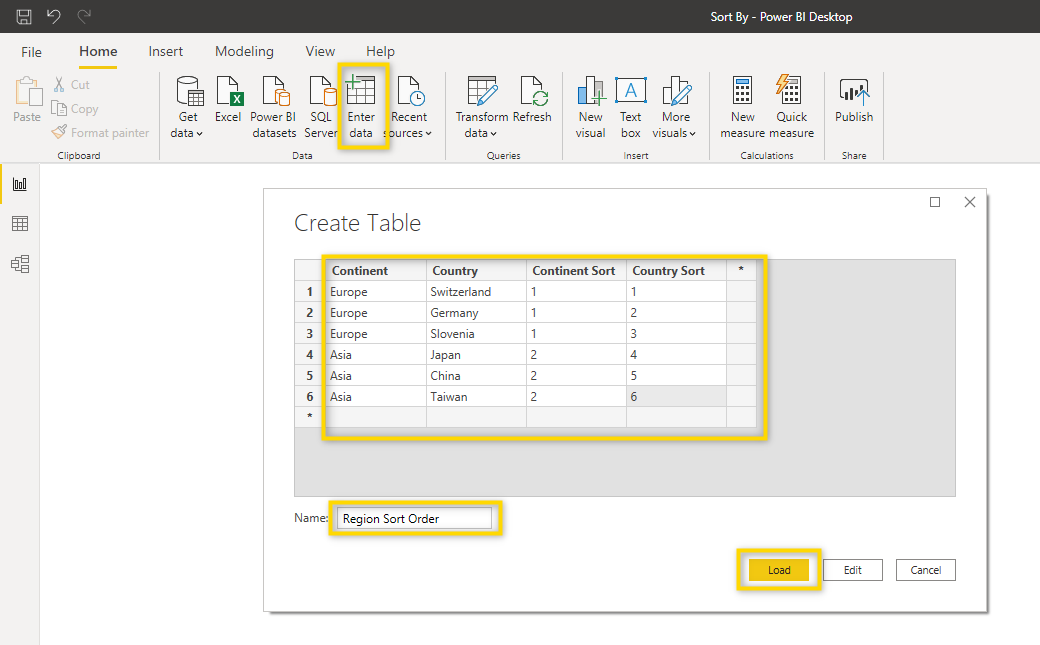Once the table has been loaded, go to the data view, select the table to which you want to add the sort order logic, and select New Column. Then you need to add the DAX function explained below.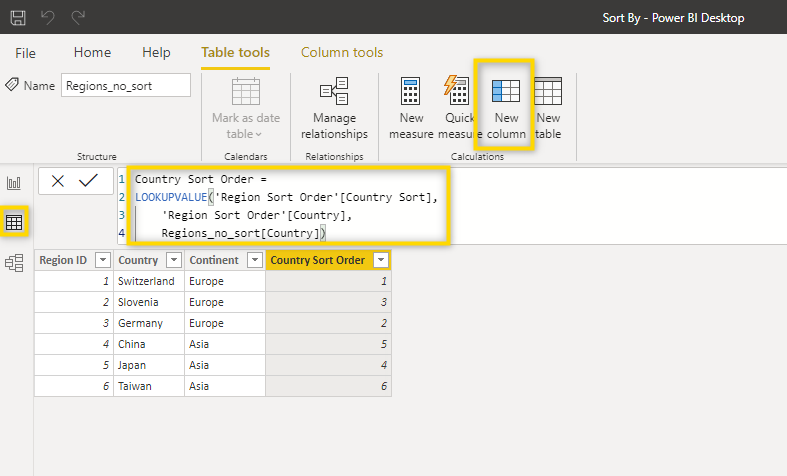```Country Sort Order =
LOOKUPVALUE('Region Sort Order'[Country Sort],
Region Sort Order'[Country],
Regions_no_sort[Country])
```

Let's break down this formula:

```Country Sort Order =
```

This is the name of the new column.

```LOOKUPVALUE('Region Sort Order'[Country Sort],
'Region Sort Order'[Country],
Regions_no_sort[Country])
```
The DAX function LOOKUPVALUE is very similar to Excel's VLOOKUP. The first argument links to the column which you want to add to the table. The second and third arguments both refer to the column Country. This is where you make the link because both tables contain the names of the countries. You first enter the column of the table with the result and then the table where it should be added.